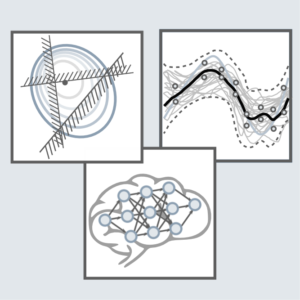# Learning in ControlAlgorithms of artificial intelligence and machine learning are of increasing importance for control engineering. At the Chair of Automatic Control, research is mainly directed towards the extension of control methods by learning components.

##### Contact

Prof. Dr.-Ing. Knut Graichen
Tel.: +49 9131 85-27127
E-Mail | Homepage

## Learning in Model Design and Identification

Regression methods allow to approximate an unknown function from individual data points. For this purpose, Gaussian process regression uses a mean function that describes the prior knowledge and a covariance function that describes the correlation between data points. The advantage with regard to other regression methods is that by learning the covariance a direct measure of the model accuracy is obtained and thus the reliability of predictions can be assessed.

In the context of control engineering, Gaussian process regression can be used in a variety of ways. One focus of research is the identification of systems, where physical modelling is either poor or requires high effort. Moreover, such learned models can be adapted online in order to reflect effects of aging or wear. A particular challenge is the real-time capable implementation, for which the number of data points must be suitably limited.

If the model includes information about the reliability, this can be used, for example, in a stochastic model predictive controller. In addition to the mean value, it computes a prediction of the uncertainty that allows to satisfy constraints with a given probability.

## Learning in Optimization and Optimal Control

Reinforcement learning aims at obtaining an optimal control strategy from repeated interactions with the system. Specifically, for each state, the action that maximizes the expected reward is searched. Formulating this task as an optimization problem shows the conceptual similarity to model predictive control, with the difference that reinforcement learning does not require model knowledge of the system.

Inverse optimal control searches a cost functional, such that the solution of the corresponding optimization problem replicates a desired system behaviour as closely as possible. This allows, for example, to use expert knowledge for the automatic determination of weighting factors for a model predictive controller.

Although many technical tasks can be formulated as optimization problems, there are cases, where the cost function or constraints can only be evaluated by costly numerical simulations. Here, Bayesian optimization allows to solve the complex optimization problem using a limited number of evaluations of the cost function and the constraints. To this end, methods  like Monte Carlo simulation or the approximation of unknown functions by Gaussian process regression can be used.